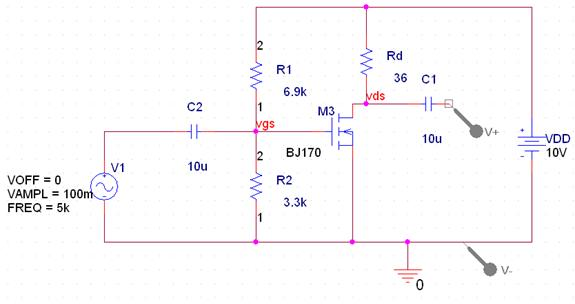Peter Lau

Bill Chun Wai Hung

Lab 12 Report – NMOS Common Source Amplifier

3 August 2004

A. Describe the Set-up

The Circuit setup is shown in figure 1.Figure 1

B1. Describe Inputs – Ideal Case

Stimuli:

vi:

Sinusoidal

Amplitude 100mv

K = 0.82

VT = 2.9 V

Vdd = 10 V

Av = 20

MOSFET = NMOS = Model BS170

C1 = C2 = 10uF.

Av= gm(Rd)

Av = 2K(VgsVto)(Rd) …(1)

Id(Rd) = 5V

K(VgsVto)^2 (Rd) = 5V … (2)

Solving (1) and (2)

Av = 2K(VgsVto)(Rd) … (1)

20 = 2(0.82)(Vgs – 2.9) (Rd) … (3)

K(VgsVto)^2 (Rd) = 5V … (2)

(0.82)(Vgs – 2.9)^2(Rd) = 5V …(4)

Solving (3) and (4)

Vgs = 3.40V

Rd = 24.4 ohm

Substitue Vgs = 3.40V

Id = K(VgsVto)^2* …(5)

Id = (0.82)(3.4 – 2.9)^2

Id = 205mA.

Vds = VddV(Rd) = 10V – 0.205 x 24.4

Vds = 5.0V

In other words, the Ideal Results are

Id=205mA.

Rd = 24.4 ohm

Vds =5.0V

Av = 20.1

B2. Describe Inputs – Experimental Case

The setup of the Experimental Case is the same as that of the Ideal case, expect

R1=6.9k

R2=3.3k

Rd = 36

The experimental circuit setup is shown in Figure 2.Figure 2

Therefore

Vgs=Vdd(R2/(R1+R2))

Vgs=10V(3.3k/(6.9k+3.3k))

Vgs = 3.24V

From (3)

20 = 2(0.82)(Vgs – 2.9) (Rd)

(20/(2*0.82)) = (Vgs – 2.9) (Rd)

12.2= (3.24 – 2.9) (Rd)

Rd = 35.9 ohm, for which, a 36 ohm resistor is used.

From (5)

Id = K(VgsVto)^2 …(5)

Id = (0.82)(3.24 – 2.9)^2

Id=94.8mA

V(Rd) = Id x Rd = 0.0948 x 36 = 3.40V, which is not the ideal 5V.

So Vds = Vdd-V(Rd) = 10V – 3.4V = 6.6V,

for V(Rd) is the voltage across Rd

In other words, the Expected Experimental Results are

Id=94.8mA

Rd = 36 ohm

Vds =6.6V

Av = 20

C. Describe What you Observe

The experimental Vo =Vds = 4.384V.

The input (Vin) amplitude is 100mA, which is 200mA peak to peak

Amplification (Av) = Vo/Vi = 22

The measured Id is

5.616/36ohm = 0.156A

In other words, the Actual Experimental Results are

Id=156 mA

Rd = 36 ohm

Vds =4.384V

Av = 22

D. What you can deduce

The Percentage Difference of the Amplification

=|Target Value – Experimental Value|/Target Value x 100%

=|20 – 22|/20 x 100%

=10%

The Percentage Difference of the Id

=|Target Value – Experimental Value|/Target Value x 100%

=|94.8mA –156mA|/94.8mA x 100%

=64.6%

The Percentage Difference of the Vds

=|Target Value – Experimental Value|/Target Value x 100%

=|6.6V –4.384V|/6.6V x 100%

=33.6%

The Experimental values are off from the calculated value by the percentages shown above. The error maybe caused by the non-perfect transistor or non-perfect resistors.

Q1) Qualitatively, describe what happens if Rd increase.

If Rd Increase, then Vds decreases. Because Vds decreases, so Vo decrease, and the amplification decreases.

Q2)Qualitatively, describe what happens if R2 decreases.

If R2 decrease, then Id decreases. Since Id decreases, V(Rd) decreases, and Vds increases, so the Vo is increased, so the amplification increased.